Electric Fields and Forces AP Physics 1 Gallup

• Slides: 53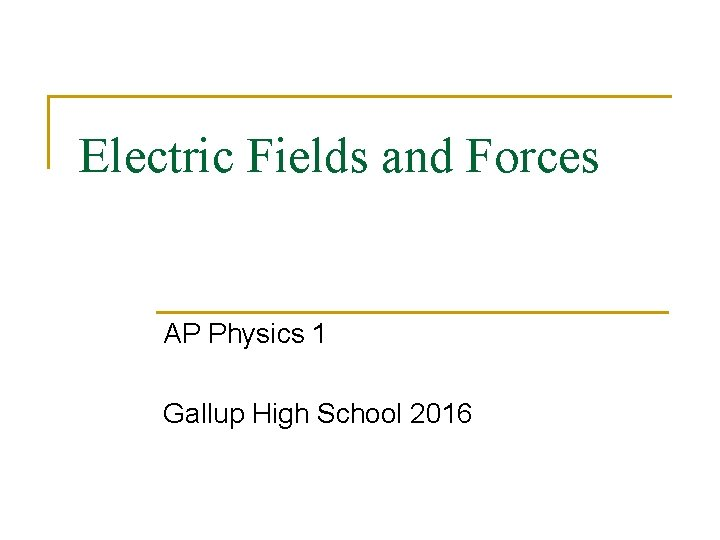Electric Fields and Forces AP Physics 1 Gallup High School 2016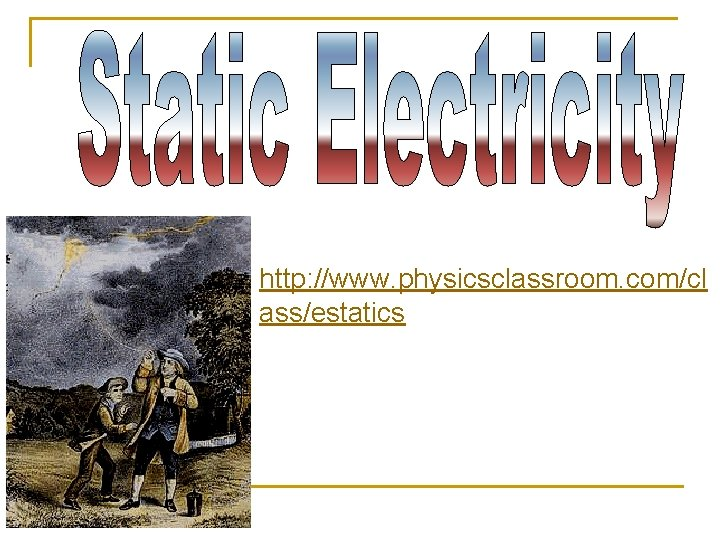http: //www. physicsclassroom. com/cl ass/estatics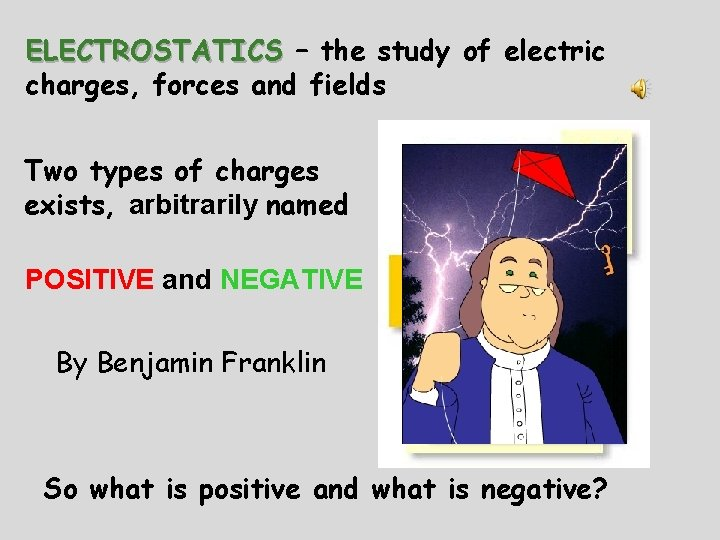ELECTROSTATICS – the study of electric charges, forces and fields Two types of charges exists, arbitrarily named POSITIVE and NEGATIVE By Benjamin Franklin So what is positive and what is negative?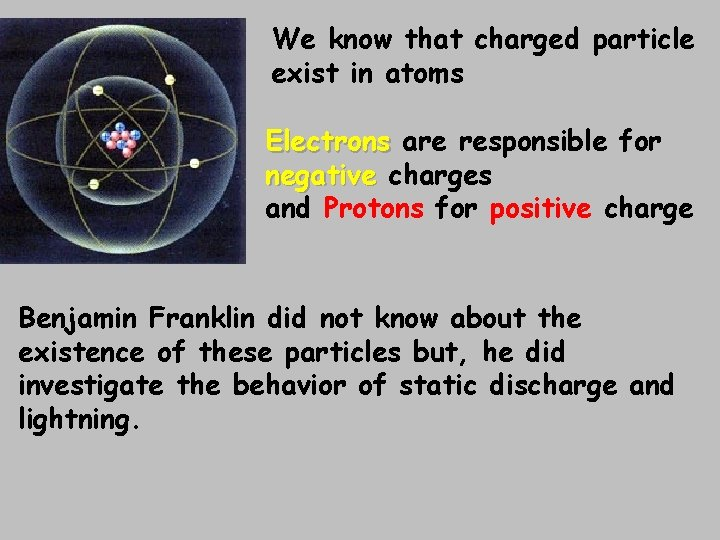We know that charged particle exist in atoms Electrons are responsible for negative charges and Protons for positive charge Benjamin Franklin did not know about the existence of these particles but, he did investigate the behavior of static discharge and lightning.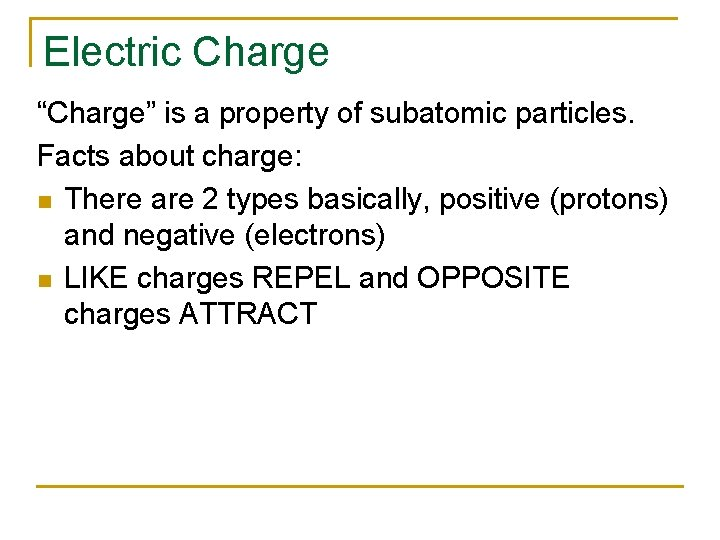Electric Charge “Charge” is a property of subatomic particles. Facts about charge: n There are 2 types basically, positive (protons) and negative (electrons) n LIKE charges REPEL and OPPOSITE charges ATTRACT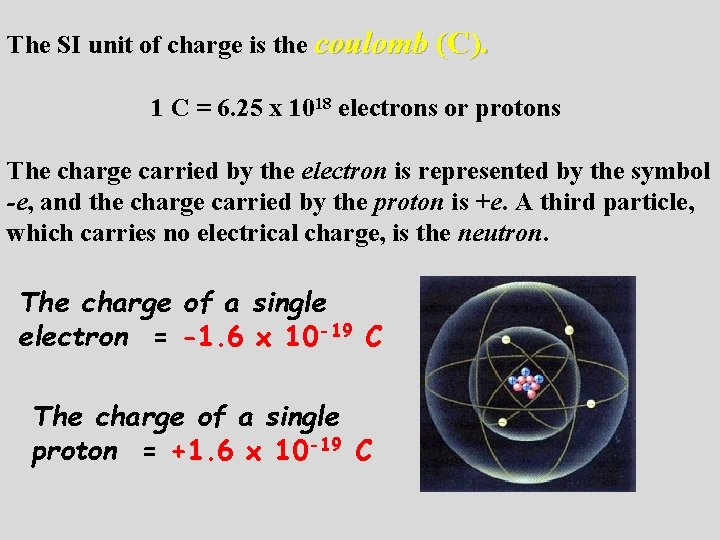The SI unit of charge is the coulomb (C). 1 C = 6. 25 x 1018 electrons or protons The charge carried by the electron is represented by the symbol -e, and the charge carried by the proton is +e. A third particle, which carries no electrical charge, is the neutron. The charge of a single electron = -1. 6 x 10 -19 C The charge of a single proton = +1. 6 x 10 -19 C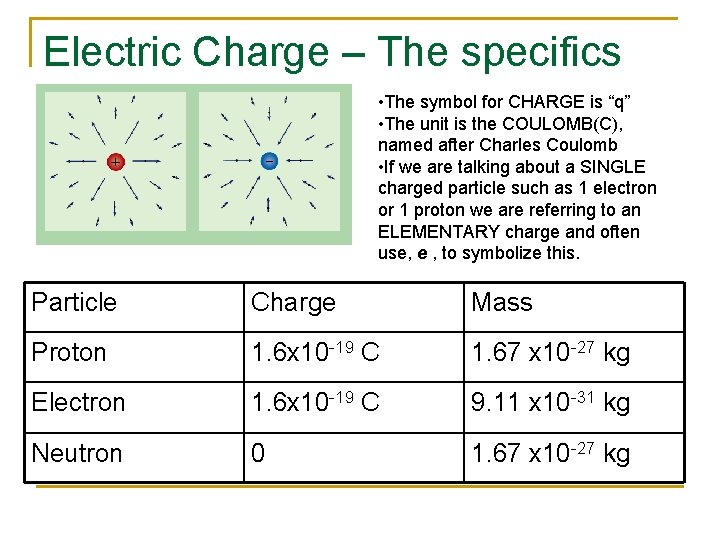Electric Charge – The specifics • The symbol for CHARGE is “q” • The unit is the COULOMB(C), named after Charles Coulomb • If we are talking about a SINGLE charged particle such as 1 electron or 1 proton we are referring to an ELEMENTARY charge and often use, e , to symbolize this. Some important constants: Particle Charge Mass Proton 1. 6 x 10 -19 C 1. 67 x 10 -27 kg Electron 1. 6 x 10 -19 C 9. 11 x 10 -31 kg Neutron 0 1. 67 x 10 -27 kgExample 1. If 16 million electrons are removed from a neutral sphere, what is the charge on the sphere in coulombs? 1 electron: e- = -1. 6 x 10 -19 C + + + + q = -2. 56 x 10 -12 C Since electrons are removed, the charge remaining on the sphere will be positive. Final charge on sphere: q = +2. 56 p. CConductors and Insulators The movement of charge is limited by the substance the charge is trying to pass through. There are generally 2 types of substances. Conductors: Allow charge to move readily though it. Insulators: Restrict the movement of the charge Conductor = Copper Wire Insulator = Plastic sheath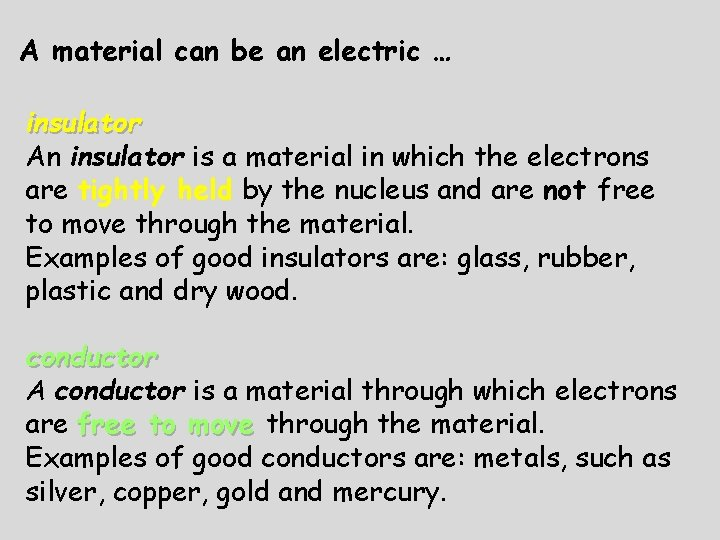A material can be an electric … insulator An insulator is a material in which the electrons are tightly held by the nucleus and are not free to move through the material. Examples of good insulators are: glass, rubber, plastic and dry wood. conductor A conductor is a material through which electrons are free to move through the material. Examples of good conductors are: metals, such as silver, copper, gold and mercury.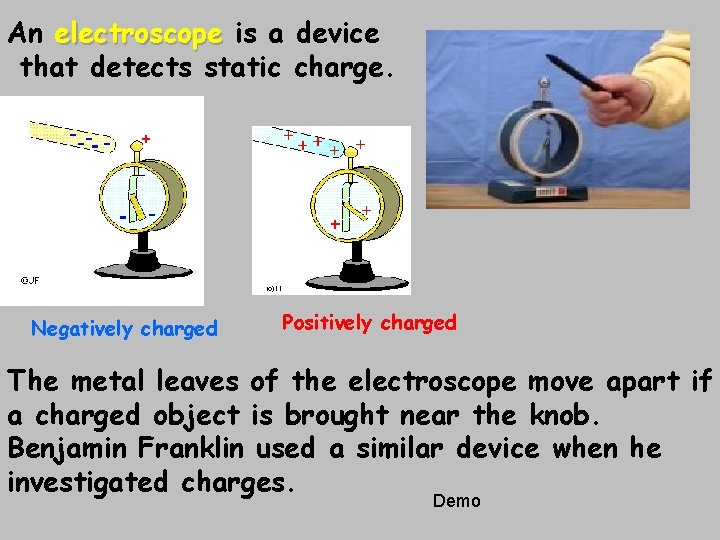An electroscope is a device that detects static charge. Negatively charged Positively charged The metal leaves of the electroscope move apart if a charged object is brought near the knob. Benjamin Franklin used a similar device when he investigated charges. Demo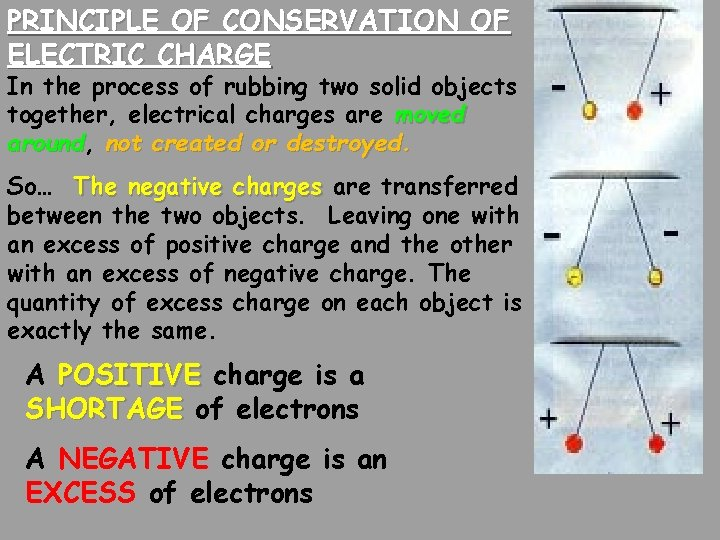PRINCIPLE OF CONSERVATION OF ELECTRIC CHARGE In the process of rubbing two solid objects together, electrical charges are moved around, around not created or destroyed. So… The negative charges are transferred between the two objects. Leaving one with an excess of positive charge and the other with an excess of negative charge. The quantity of excess charge on each object is exactly the same. A POSITIVE charge is a SHORTAGE of electrons A NEGATIVE charge is an EXCESS of electronsCharge is “CONSERVED” Charge cannot be created or destroyed only transferred from one object to another. Even though these 2 charges attract initially, they repel after touching. Notice the NET charge stays the same.Objects become charged by… Friction Electrons are rubbed off one insulator on to another insulator Induction Charging an object WITHOUT touching a charged object Charging by CONTACT with a Conduction charged object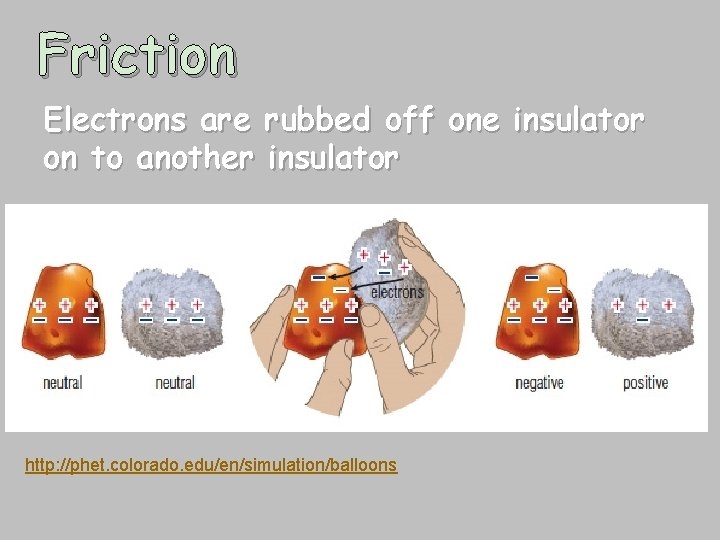Friction Electrons are rubbed off one insulator on to another insulator http: //phet. colorado. edu/en/simulation/balloonsWhen a glass rod is rubbed against silk, electrons are removed from the glass and deposited on the silk. Electrons move from glass to the silk cloth. glass positive negative silk - - + + + + The glass is said to be positively charged because of a deficiency of electrons. The silk is said to be negatively charged because of an excess of electrons.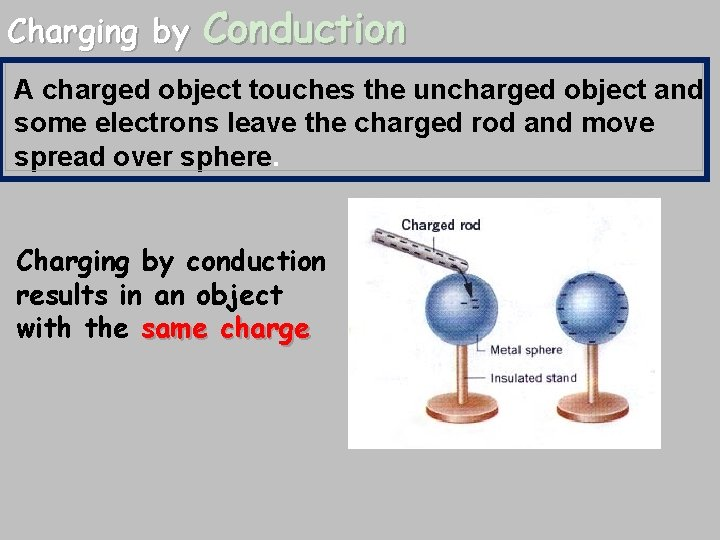Charging by Conduction A charged object touches the uncharged object and some electrons leave the charged rod and move spread over sphere. Charging by conduction results in an object with the same charge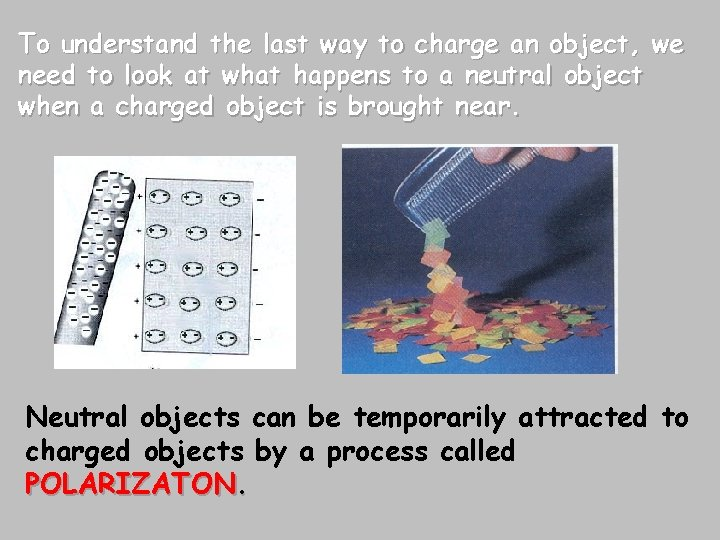To understand the last way to charge an object, we need to look at what happens to a neutral object when a charged object is brought near. Neutral objects can be temporarily attracted to charged objects by a process called POLARIZATON.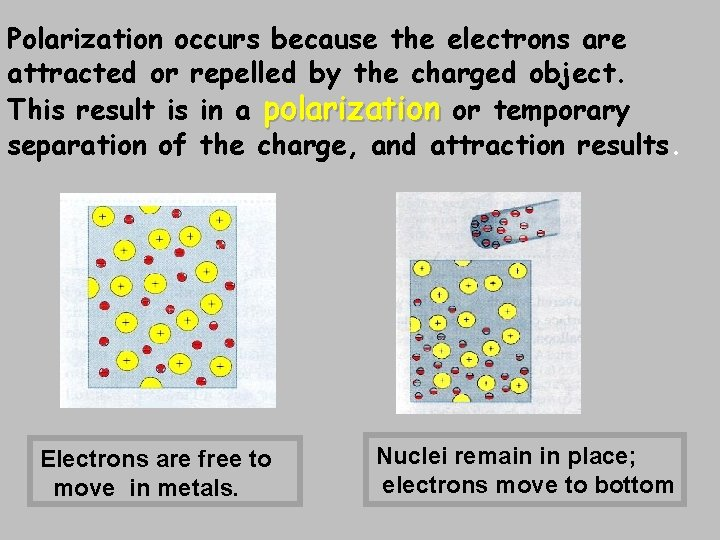Polarization occurs because the electrons are attracted or repelled by the charged object. This result is in a polarization or temporary separation of the charge, and attraction results. Electrons are free to move in metals. Nuclei remain in place; electrons move to bottomCharging by Induction polarization grounding permanent charge The rod does not touch the sphere. It pushes electrons out of the back side of the sphere and down the wire to ground. The ground wire is disconnected to prevent the return of the electrons from ground, then the rod is removed. The resulting charge on the object is oppositeInduction and Grounding The second way to charge something is via INDUCTION, which requires NO PHYSICAL CONTACT. We bring a negatively charged rod near a neutral sphere. The protons in the sphere localize near the rod, while the electrons are repelled to the other side of the sphere. A wire can then be brought in contact with the negative side and allowed to touch the GROUND. The electrons will always move towards a more massive objects to increase separation from other electrons, leaving a NET positive sphere behind.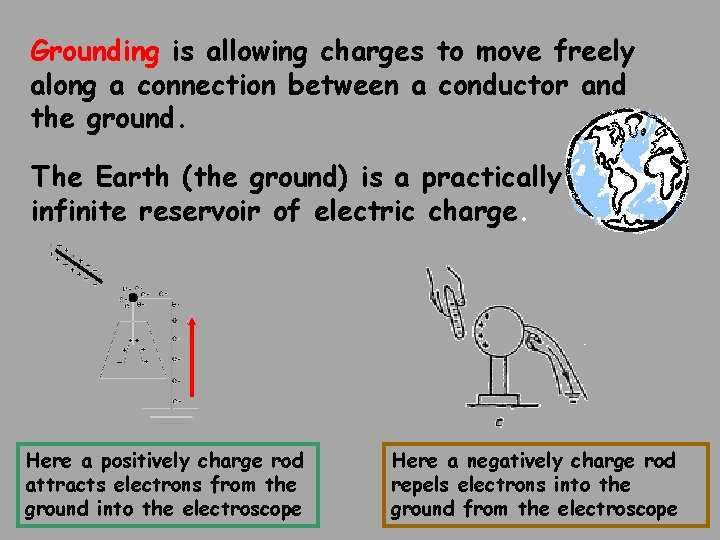Grounding is allowing charges to move freely along a connection between a conductor and the ground. The Earth (the ground) is a practically infinite reservoir of electric charge. Here a positively charge rod attracts electrons from the ground into the electroscope Here a negatively charge rod repels electrons into the ground from the electroscope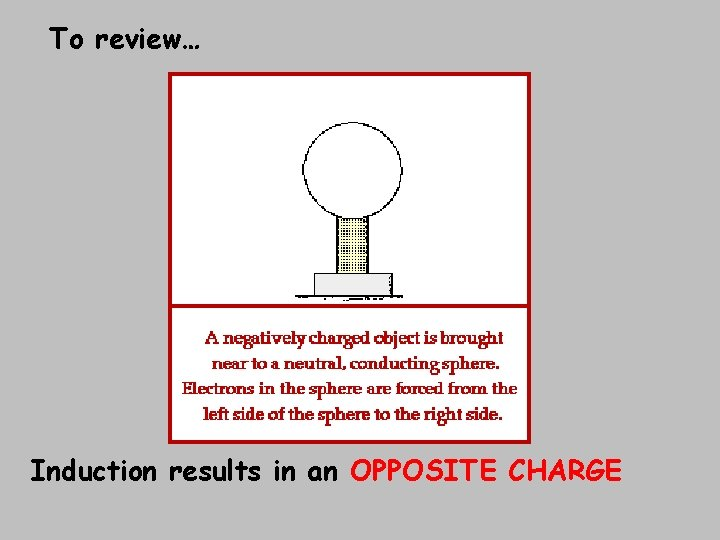To review… Induction results in an OPPOSITE CHARGE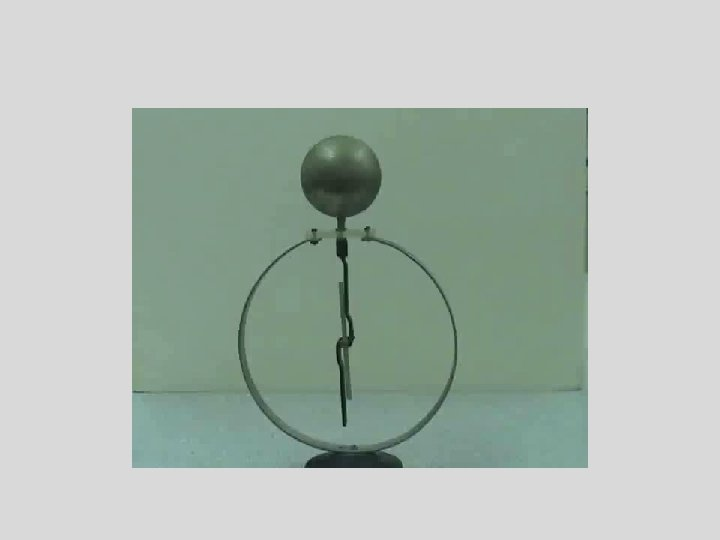Applications of Electrostatic charging Fine mist of negatively charged gold particles adhere to Negatively charged paint positively charged protein on adheres to positively fingerprint. charged metal.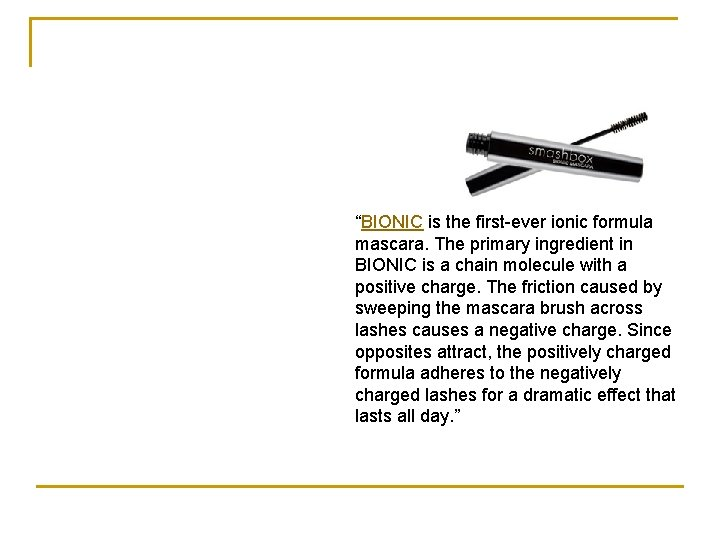“BIONIC is the first-ever ionic formula mascara. The primary ingredient in BIONIC is a chain molecule with a positive charge. The friction caused by sweeping the mascara brush across lashes causes a negative charge. Since opposites attract, the positively charged formula adheres to the negatively charged lashes for a dramatic effect that lasts all day. ”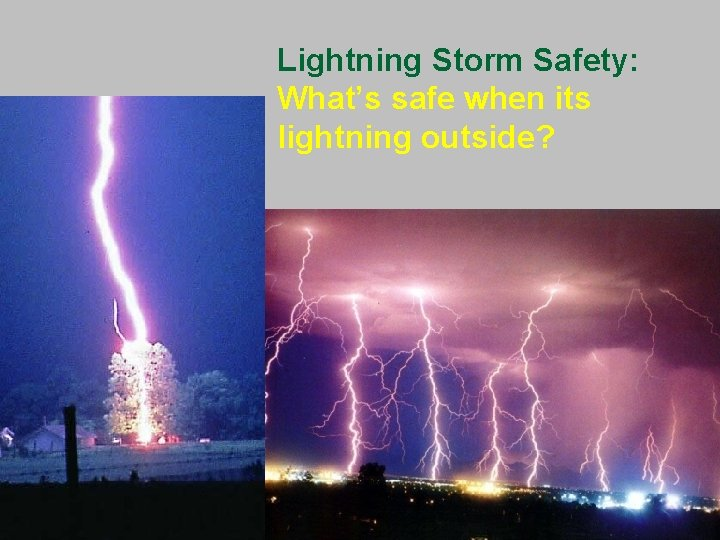Lightning Storm Safety: What’s safe when its lightning outside?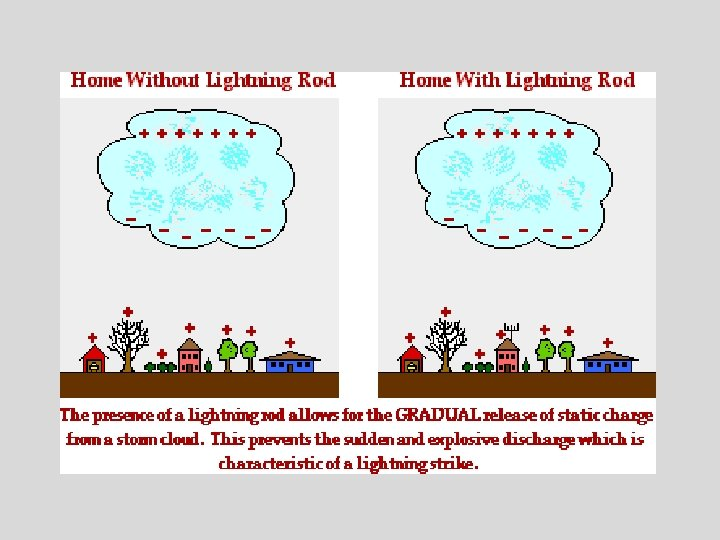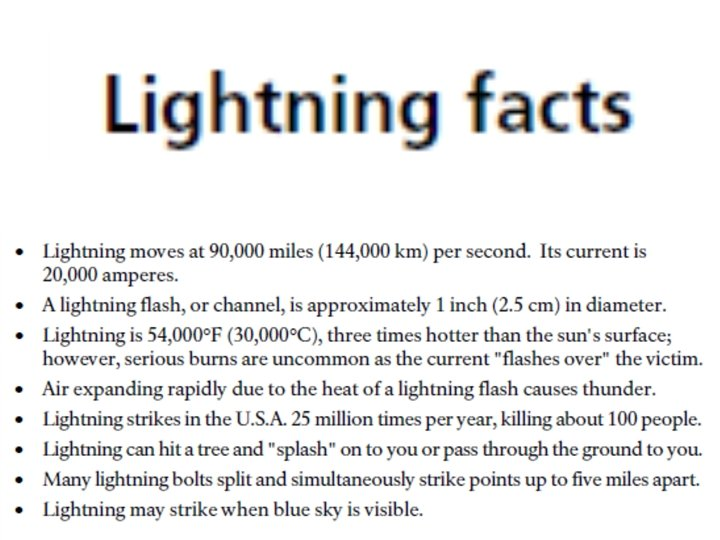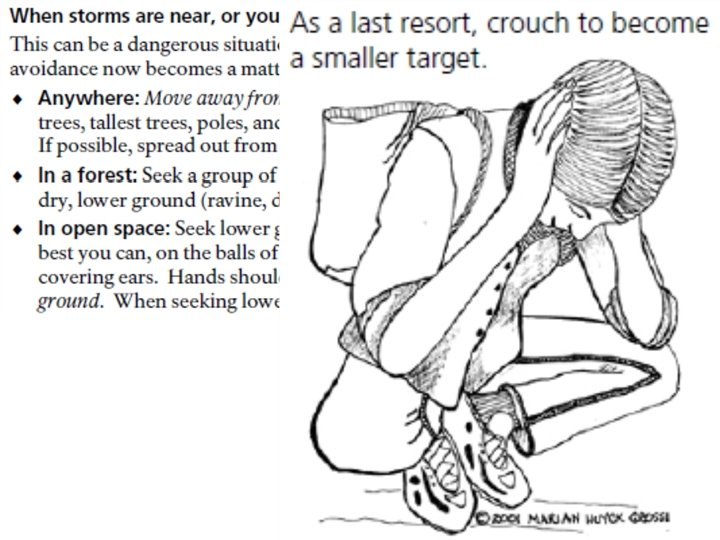Coulomb’s Law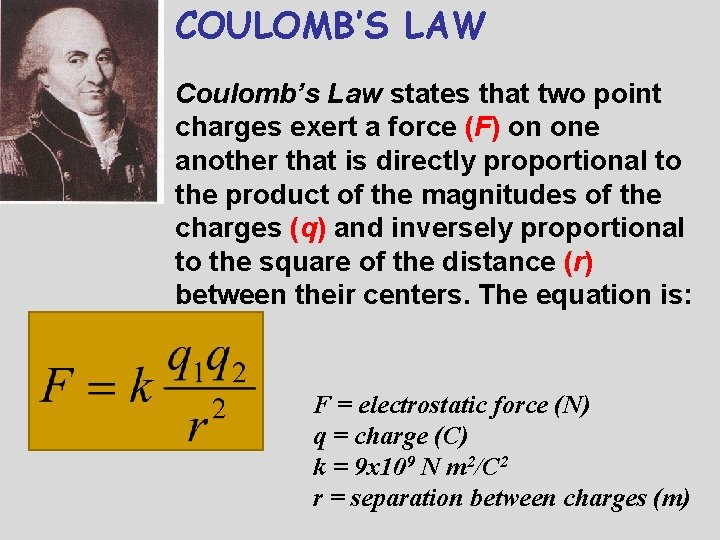COULOMB’S LAW Coulomb’s Law states that two point charges exert a force (F) on one another that is directly proportional to the product of the magnitudes of the charges (q) and inversely proportional to the square of the distance (r) between their centers. The equation is: F = electrostatic force (N) q = charge (C) k = 9 x 109 N m 2/C 2 r = separation between charges (m)The coulomb (selected for use with electric currents) is actually a very large unit for static electricity. It is common to use the metric prefixes. 1 m. C = 1 x 10 -6 C 1 n. C = 1 x 10 -9 C 1 p. C = 1 x 10 -12 CElectric Force The electric force between 2 objects is symbolic of the gravitational force between 2 objects. RECALL: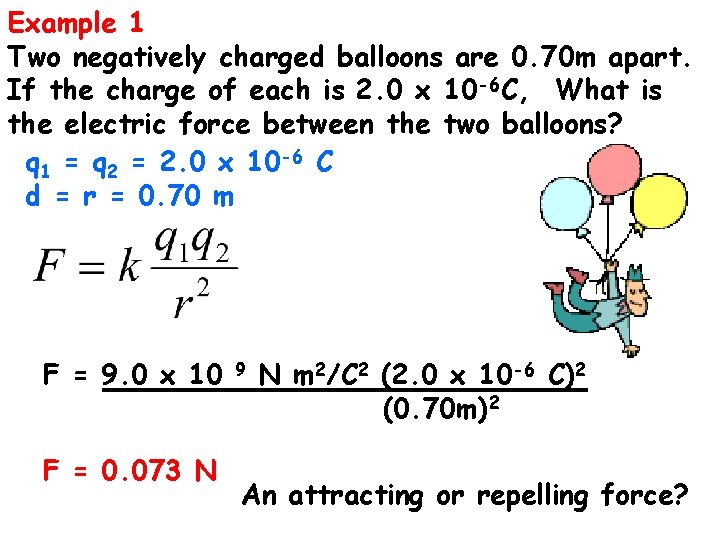Example 1 Two negatively charged balloons are 0. 70 m apart. If the charge of each is 2. 0 x 10 -6 C, What is the electric force between the two balloons? q 1 = q 2 = 2. 0 x 10 -6 C d = r = 0. 70 m F = 9. 0 x 10 F = 0. 073 N 9 N m 2/C 2 (2. 0 x 10 -6 C)2 (0. 70 m)2 An attracting or repelling force?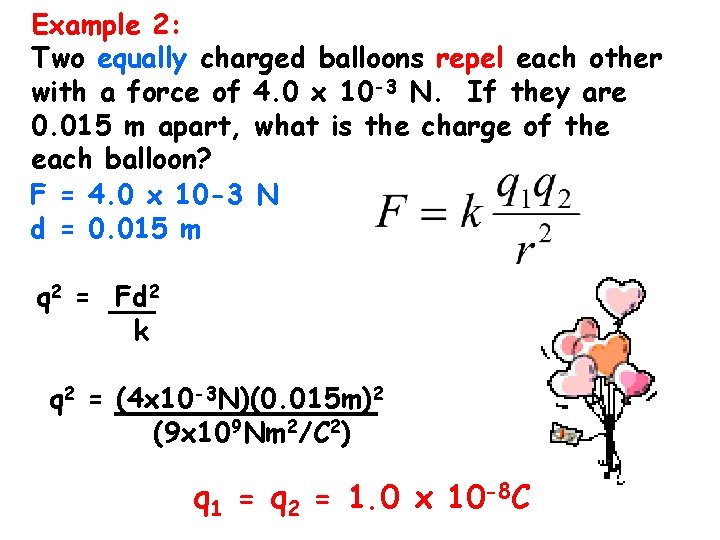Example 2: Two equally charged balloons repel each other with a force of 4. 0 x 10 -3 N. If they are 0. 015 m apart, what is the charge of the each balloon? F = 4. 0 x 10 -3 N d = 0. 015 m q 2 = Fd 2 k q 2 = (4 x 10 -3 N)(0. 015 m)2 (9 x 109 Nm 2/C 2) q 1 = q 2 = 1. 0 x 10 -8 C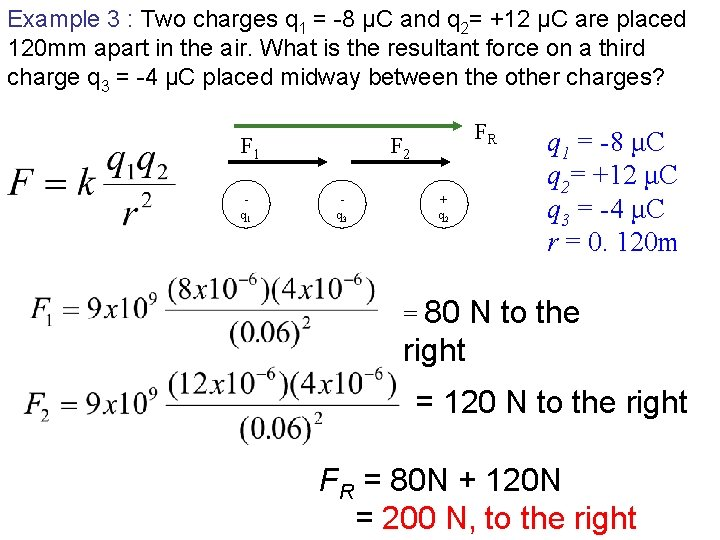Example 3 : Two charges q 1 = -8 μC and q 2= +12 μC are placed 120 mm apart in the air. What is the resultant force on a third charge q 3 = -4 μC placed midway between the other charges? F 1 FR F 2 - - + q 1 q 3 q 2 q 1 = -8 μC q 2= +12 μC q 3 = -4 μC r = 0. 120 m = 80 N to the right = 120 N to the right FR = 80 N + 120 N = 200 N, to the right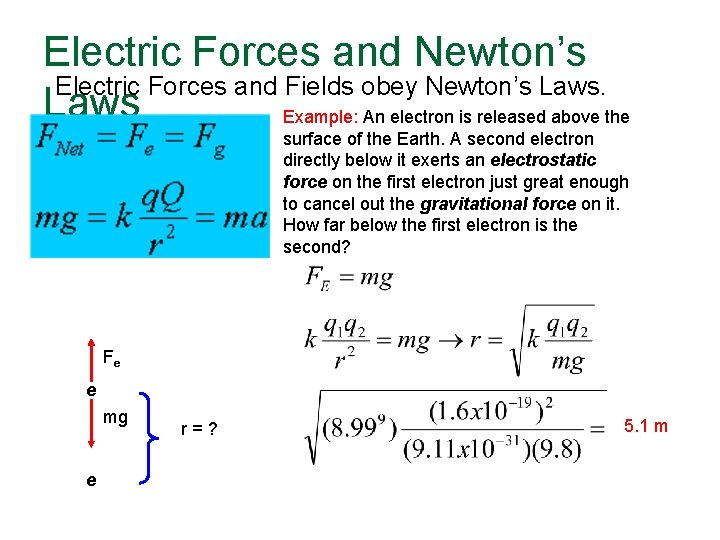Electric Forces and Newton’s Electric Forces and Fields obey Newton’s Laws Example: An electron is released above the surface of the Earth. A second electron directly below it exerts an electrostatic force on the first electron just great enough to cancel out the gravitational force on it. How far below the first electron is the second? Fe e mg e r = ? 5. 1 m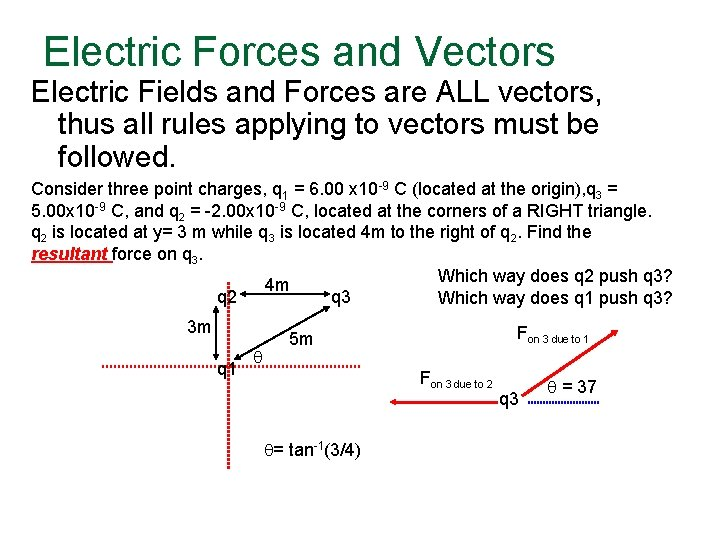Electric Forces and Vectors Electric Fields and Forces are ALL vectors, thus all rules applying to vectors must be followed. Consider three point charges, q 1 = 6. 00 x 10 -9 C (located at the origin), q 3 = 5. 00 x 10 -9 C, and q 2 = -2. 00 x 10 -9 C, located at the corners of a RIGHT triangle. q 2 is located at y= 3 m while q 3 is located 4 m to the right of q 2. Find the resultant force on q 3. Which way does q 2 push q 3? 4 m q 2 q 3 Which way does q 1 push q 3? 3 m q 1 q Fon 3 due to 1 5 m Fon 3 due to 2 q= tan-1(3/4) q 3 q = 37Example Cont’ 4 m q 2 3 m q 1 q q 3 Fon 3 due to 1 5 m q= tan-1(3/4) Fon 3 due to 2 q 3 q = 37 F 3, 1 sin 37 F 3, 1 cos 37 5. 6 x 10 -9 N 7. 34 x 10 -9 N 1. 1 x 10 -8 N 64. 3 degrees above the +xExample 4. Three charges, q 1 = +8 m. C, q 2 = +6 m. C and q 3 = -4 m. C are arranged as shown below. Find the resultant force on the – 4 m. C charge due to the others. +6 m. C 3 cm q 3 - -4 m. C q 2 + 4 cm q 1 5 cm + 53. 1 gram. a i d y d ee-bo r f f w a r D F 2 q 3 - -4 m. C 53. 1 o F 1 o +8 m. C Note the directions of forces F 1 and F 2 on q 3 based on attraction/repulsion from q 1 and q 2.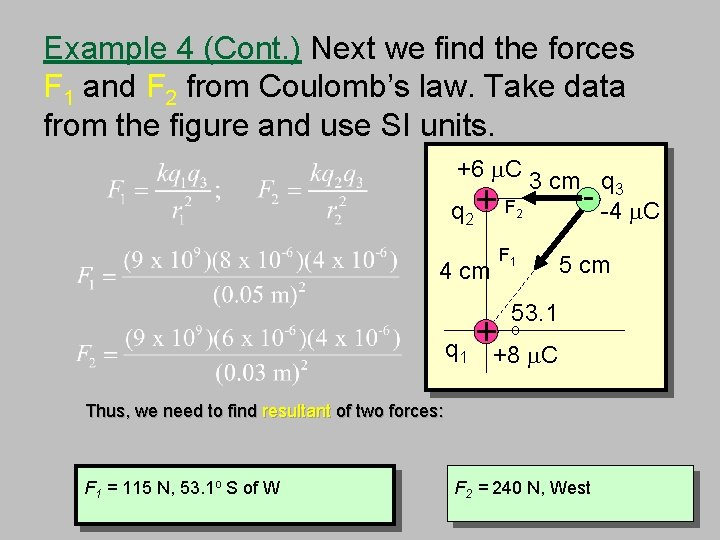Example 4 (Cont. ) Next we find the forces F 1 and F 2 from Coulomb’s law. Take data from the figure and use SI units. +6 m. C 3 cm q 3 - -4 m. C q 2 + F 2 F 1 4 cm q 1 + 5 cm 53. 1 o +8 m. C Thus, we need to find resultant of two forces: F 1 = 115 N, 53. 1 o S of W F 2 = 240 N, West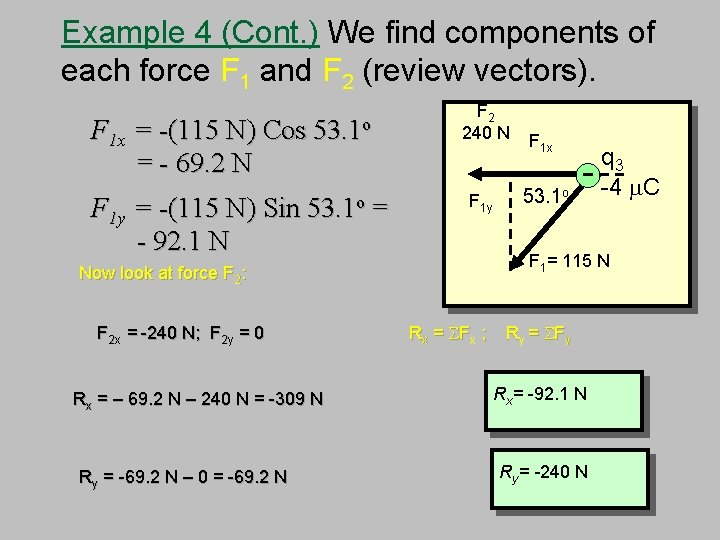Example 4 (Cont. ) We find components of each force F 1 and F 2 (review vectors). F 1 x = -(115 N) Cos = - 69. 2 N 53. 1 o F 1 y = -(115 N) Sin 53. 1 o = - 92. 1 N Now look at force F 2: F 2 x = -240 N; F 2 y = 0 Rx = – 69. 2 N – 240 N = -309 N Ry = -69. 2 N – 0 = -69. 2 N F 2 240 N F 1 y F 1 x q 3 - -4 m. C o 53. 1 F 1= 115 N Rx = SFx ; Ry = SFy Rx= -92. 1 N Ry= -240 N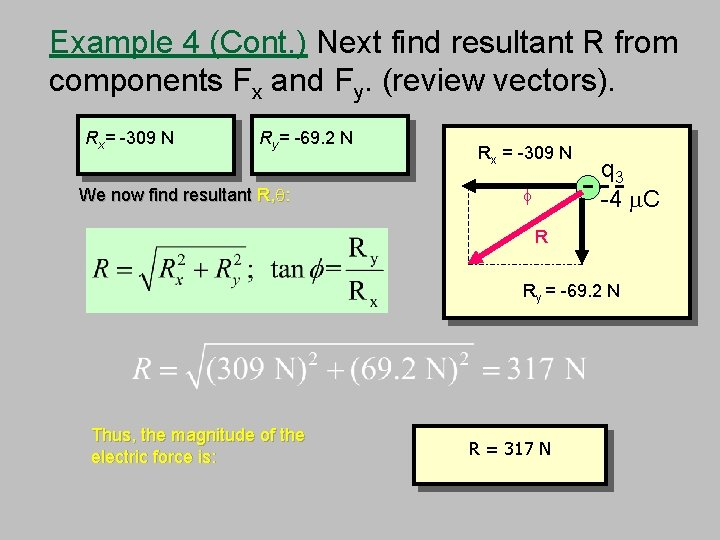Example 4 (Cont. ) Next find resultant R from components Fx and Fy. (review vectors). Rx= -309 N Ry= -69. 2 N We now find resultant R, q: Rx = -309 N f q 3 - -4 m. C R Ry = -69. 2 N Thus, the magnitude of the electric force is: R = 317 N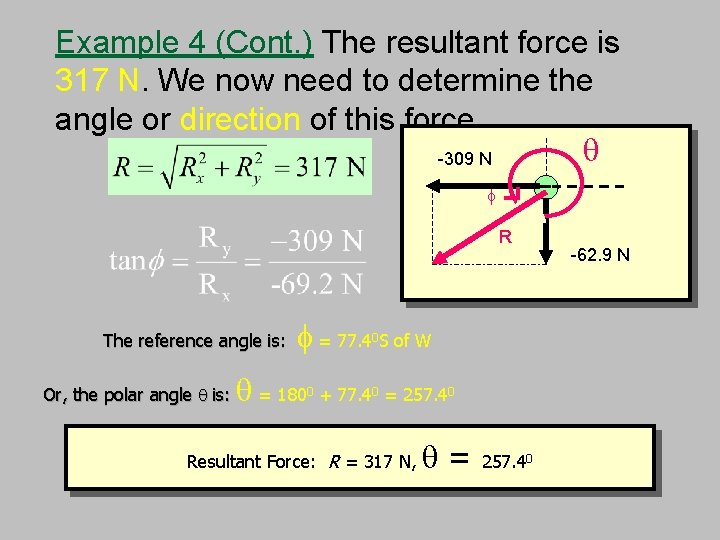Example 4 (Cont. ) The resultant force is 317 N. We now need to determine the angle or direction of this force. q -309 N f R The reference angle is: Or, the polar angle q is: f = 77. 4 S of W q = 180 0 0 + 77. 40 = 257. 40 Resultant Force: R = 317 N, q= 257. 40 -69. 2 N -62. 9 NElectric Fields By definition, they are “LINES OF FORCE” Some important facts: n An electric field is a vector n Always is in the direction that a POSITIVE “test” charge would move n The amount of force PER “test” charge If you placed a 2 nd positive charge (test charge), near the positive charge shown above, it would move AWAY. If you placed that same charge near the negative charge shown above it would move TOWARDS.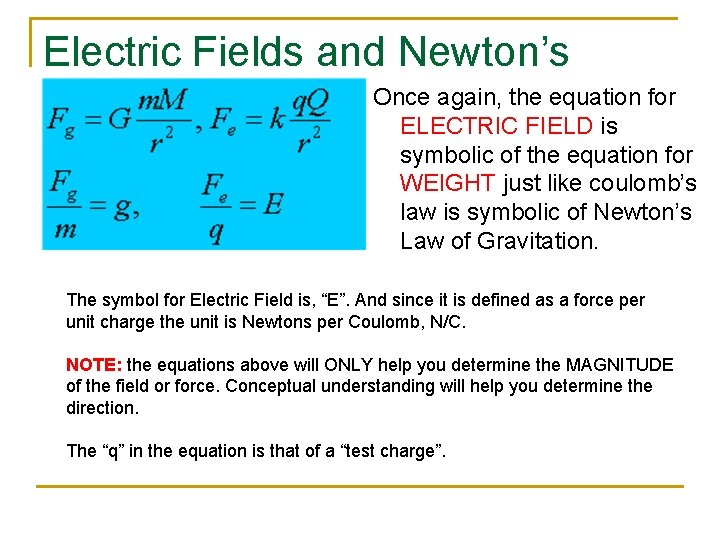Electric Fields and Newton’s Once again, the equation for Laws ELECTRIC FIELD is symbolic of the equation for WEIGHT just like coulomb’s law is symbolic of Newton’s Law of Gravitation. The symbol for Electric Field is, “E”. And since it is defined as a force per unit charge the unit is Newtons per Coulomb, N/C. NOTE: the equations above will ONLY help you determine the MAGNITUDE of the field or force. Conceptual understanding will help you determine the direction. The “q” in the equation is that of a “test charge”.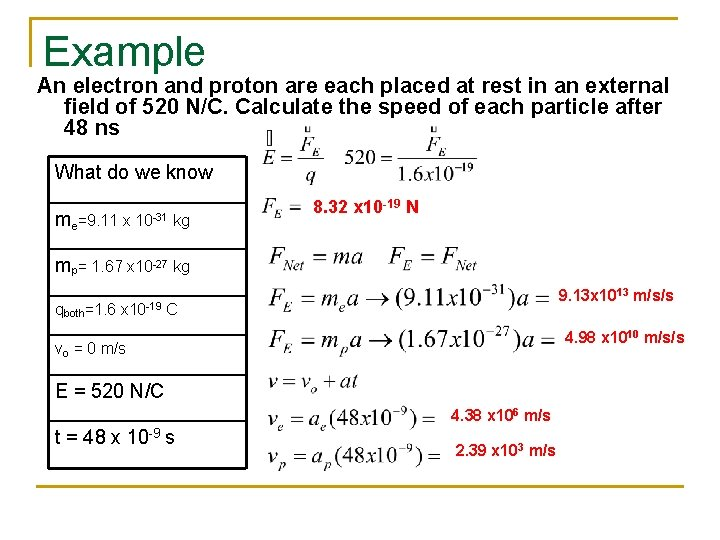Example An electron and proton are each placed at rest in an external field of 520 N/C. Calculate the speed of each particle after 48 ns What do we know me=9. 11 x 10 -31 kg 8. 32 x 10 -19 N mp= 1. 67 x 10 -27 kg 9. 13 x 1013 m/s/s qboth=1. 6 x 10 -19 C 4. 98 x 1010 m/s/s vo = 0 m/s E = 520 N/C 4. 38 x 106 m/s t = 48 x 10 -9 s 2. 39 x 103 m/sAn Electric Point Charge As we have discussed, all charges exert forces on other charges due to a field around them. Suppose we want to know how strong the field is at a specific point in space near this charge the calculate the effects this charge will have on other charges should they be placed at that point. POINT CHARGE TEST CHARGE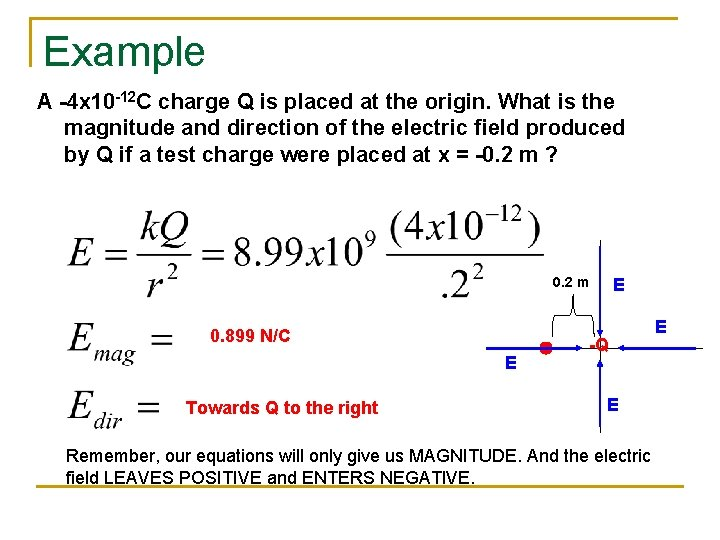Example A -4 x 10 -12 C charge Q is placed at the origin. What is the magnitude and direction of the electric field produced by Q if a test charge were placed at x = -0. 2 m ? 0. 2 m 0. 899 N/C E Towards Q to the right E -Q E E Remember, our equations will only give us MAGNITUDE. And the electric field LEAVES POSITIVE and ENTERS NEGATIVE.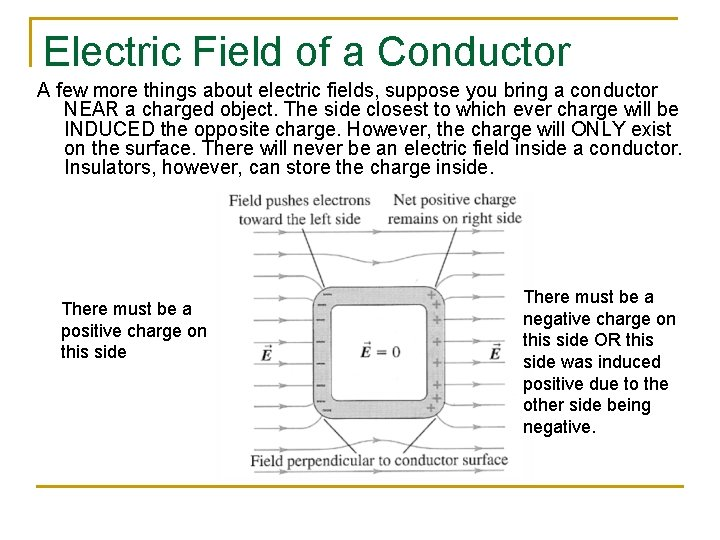Electric Field of a Conductor A few more things about electric fields, suppose you bring a conductor NEAR a charged object. The side closest to which ever charge will be INDUCED the opposite charge. However, the charge will ONLY exist on the surface. There will never be an electric field inside a conductor. Insulators, however, can store the charge inside. There must be a positive charge on this side There must be a negative charge on this side OR this side was induced positive due to the other side being negative.